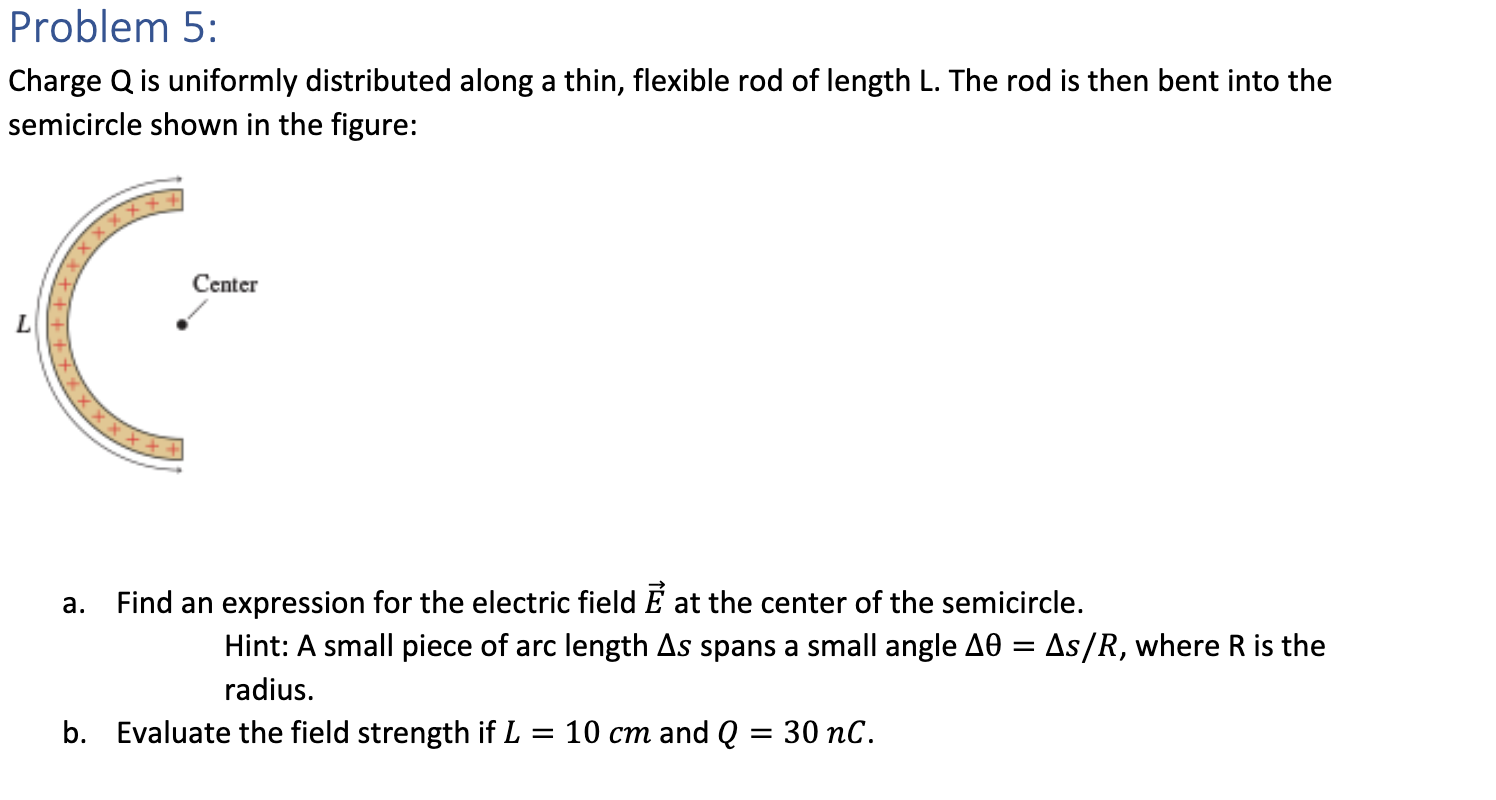# Question Solved1 AnswerProblem 5: Charge Q is uniformly distributed along a thin, flexible rod of length L. The rod is then bent into the semicircle shown in the figure: Center L C a. Find an expression for the electric field at the center of the semicircle. Hint: A small piece of arc length As spans a small angle 10 = As/R, where R is the radius. b. Evaluate the field strength if L = 10 cm and Q = 30 nC.Transcribed Image Text: Problem 5: Charge Q is uniformly distributed along a thin, flexible rod of length L. The rod is then bent into the semicircle shown in the figure: Center L C a. Find an expression for the electric field at the center of the semicircle. Hint: A small piece of arc length As spans a small angle 10 = As/R, where R is the radius. b. Evaluate the field strength if L = 10 cm and Q = 30 nC.
More
Transcribed Image Text: Problem 5: Charge Q is uniformly distributed along a thin, flexible rod of length L. The rod is then bent into the semicircle shown in the figure: Center L C a. Find an expression for the electric field at the center of the semicircle. Hint: A small piece of arc length As spans a small angle 10 = As/R, where R is the radius. b. Evaluate the field strength if L = 10 cm and Q = 30 nC.